# Variable gain superbal circuit preserves CMRR

## Texas Instruments NE5532

Merlin Blencowe

EDN

The superbal circuit will be familiar to anyone involved in balanced signal transmission [1,2]; it is a differential amplifier which presents identical input impedances to both legs of a balanced line. Balanced loading is a necessity for the switching systems used in some audio mixing consoles, for example, to ensure signal balance and common mode rejection ratio (CMRR) are always maintained.

The archetypal superbal circuit is shown in Figure 1, where it is always assumed that Z1 = Z5, Z2 = Z6, and Z3 = Z4. U1 is a familiar differential amplifier while U2 is used to drive the bottom of Z6 with an equal-but-opposite signal to the output, so that a virtual earth is maintained at both of U1’s input terminals, and the input impedance is equal to Z1 and Z5 for both balanced legs. The voltage gain is set according to the ratio of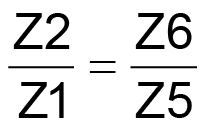in the usual way for a differential amplifier.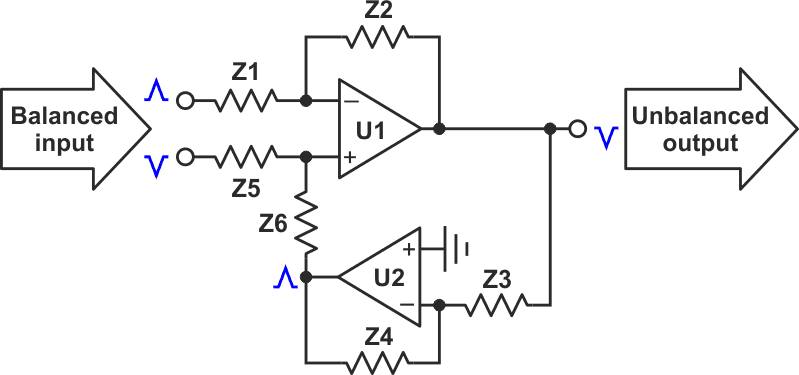Figure 1. U1 and U2 were implemented with a NE5532 dual op amp, but this circuit works with nearly any similar device.

However, difficulty arises if we need the gain to be adjustable since both arms must be varied simultaneously and identically; even a small difference between them will lead to rapid degradation of CMRR. A solution to this problem is shown in Figure 2.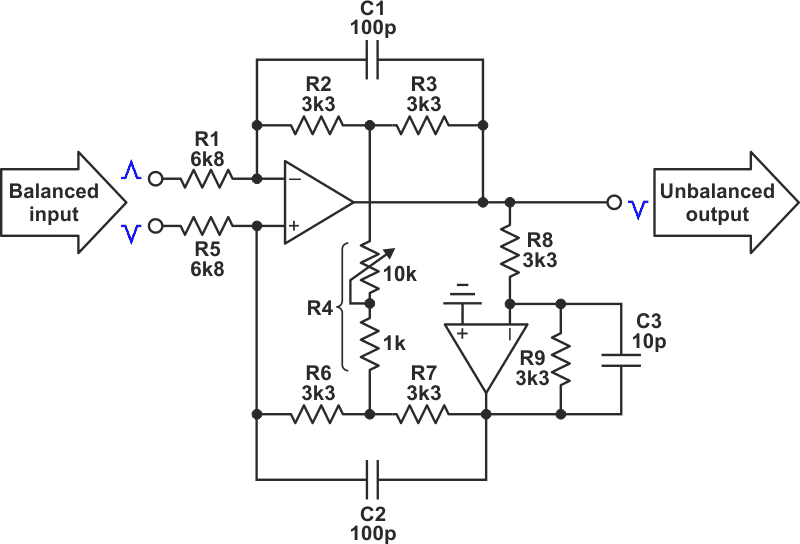Figure 2. This solution allows for adjustable gain.

Here Z2 and Z6 have been broken into two equal parts formed by R2-3 and R6-7 respectively, and a bridging resistance R4 is connected between their junctions. A virtual earth exists at the centre of R4, which means the feedback factor of U1 can be adjusted, but without upsetting the circuit symmetry. CMRR is therefore not degraded and an ordinary variable resistor can be used (close tolerance resistors must still be used for R1-R9, as for any differential amplifier).

Assuming always that R1 = R5 and R2 = R3 = R6 = R7 and R8 = R9, the differential voltage gain is equal to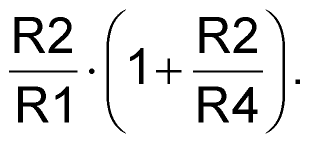The component values shown have been selected to give a useful range of trim from about –4 dB to +6 dB, but this can be altered to suit the application, of course.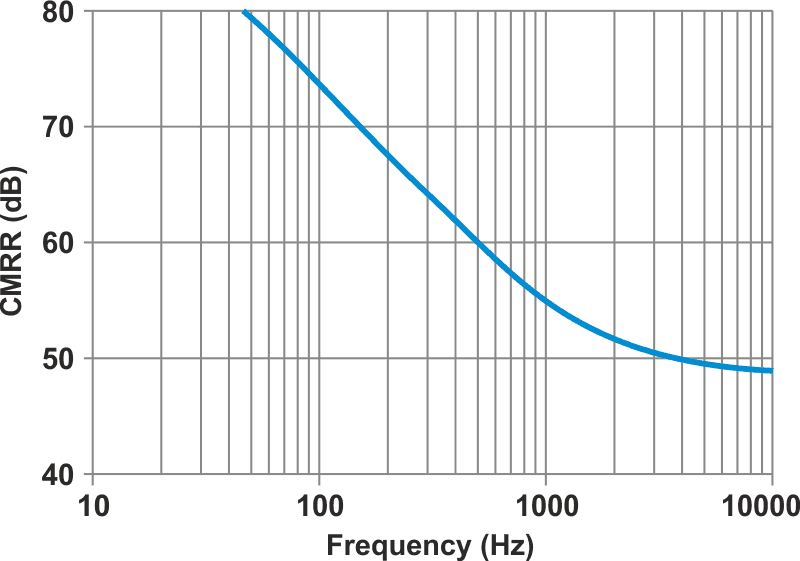Figure 3. This plot shows the measured CMRR with a dual op amp and unselected 1% resistors used throughout.

The plot in Figure 3 illustrates the measured CMRR with an NE5532 dual op amp and unselected 1% resistors used throughout; this performance was unchanged at all gain settings. This adaptation of the superbal allowed the author to incorporate a gain-trim into a mixing console where space was already too limited for another op amp.

EDN